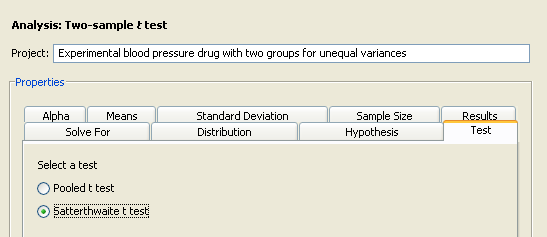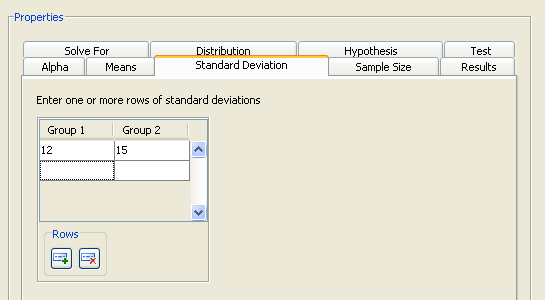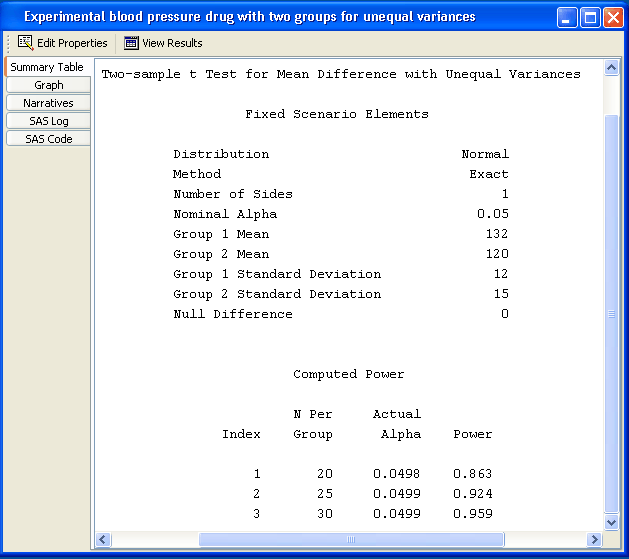Test of Two Independent Means for Unequal Variances

In the preceding example, you assumed that the population standard deviations were equal. If you believe that the population standard deviations are not equal, use the same two-sample t test analysis as with the preceding example, but change the test option and enter group standard deviations.

You can use the previous example to demonstrate this test. If the project is not already open, open it by selecting FileOpen on the menu bar, and then selecting the project that you have been using.

Make a copy of the project by selecting FileSave As. Enter a different project description, Experimental blood pressure drug with two groups for unequal variances. Click OK.

The copy of the project is opened, and the current project is closed.

### Editing Properties

#### Test

On the Test tab of the copied project, change the test to Satterthwaite t test, as shown in Figure 71.46.

Figure 71.46 Satterthwaite t Test Option#### Specifying Group Standard Deviations

Click the Standard Deviation tab and enter the group standard deviations of 12 and 15 on a single row, as shown in Figure 71.47.

Figure 71.47 Group Standard Deviations#### Summary of Input Parameters

Table 71.3 contains the values of the input parameters for the example.

Table 71.3 Summary of Input Parameters

Parameter

Value

Distribution

Normal

Hypothesis

One-sided test

Test

Satterthwaite t test

Alpha

0.05

Means form

Group means

Means

132, 120

Standard deviation

12, 15

Sample size form

Sample size per group

Sample size

20, 25, 30

Click Calculate to run the analysis.

### Viewing Results

The power for a sample size per group of 25 is 0.924, as shown in Figure 71.48. Notice that the actual alpha is 0.0499. This is because the Satterthwaite t test is (slightly) biased.

Figure 71.48 Satterthwaite Test ResultsIf you modified the previous example, when you select the Narratives tab, the following message is displayed:

Previously selected narratives have been cleared because one or more input parameter values have changed.

In the previous analysis, you created narratives for two scenarios. Because this analysis uses group standard deviations, those selected narratives were cleared. The message would also have appeared if you had changed the number of scenarios.

Use the narrative selector table to create other narratives.